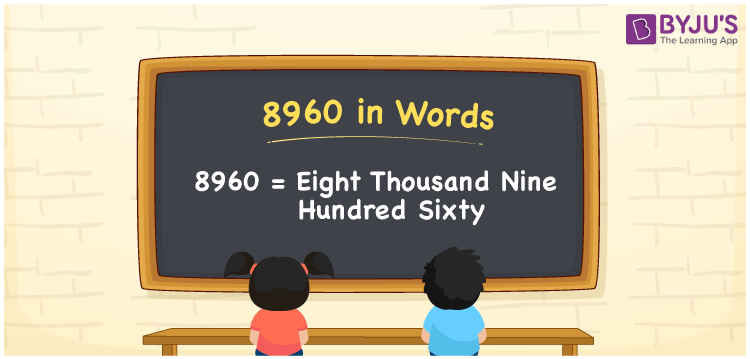# 8960 in Words

8960 in words is written as Eight thousand nine hundred sixty. In both the International System of Numerals and the Indian System of Numerals, 8960 is written as Eight thousand nine hundred sixty. The number 8960 is a Cardinal Number as it represents some quantity. For example, “that crystal wares cost 8960 rupees”.

 8960 in Words Eight thousand nine hundred sixty Eight thousand nine hundred sixty in Number 8960

## 8960 in English Words

8960 in English words is read as “Eight thousand nine hundred sixty”.## How to Write 8960 in Words?

To write 8960 in words, we shall use the place value chart. In the place value chart, put 8 in the thousands, 9 in the hundreds, 6 in the tens, and 0 in the ones, respectively. Let us make a place value chart to write the number 8960 in words.

 Thousands Hundreds Tens Ones 8 9 6 0

Thus, we can write the expanded form as

8 × Thousand + 9 × Hundred + 6 × Ten + 0 × One

= 8 × 1000 + 9 × 100 + 6 × 10 + 0 × 1

= 8000 + 900 + 60 + 0

= 8960

= Eight thousand nine hundred sixty.

8960 is a natural number that is the successor of 8959 and the predecessor of 8961.

8960 in words – Eight thousand nine hundred sixty

• Is 8960 an odd number? – No
• Is 8960 an even number? – Yes
• Is 8960 a perfect square number? – No
• Is 8960 a perfect cube number? – No
• Is 8960 a prime number? – No
• Is 8960 a composite number? – Yes

## Frequently Asked Questions on 8960 in Words

Q1

### How to write 8960 in words?

8960 in words is written as Eight thousand nine hundred sixty.
Q2

### How to write 8960 in the International and Indian System of Numerals?

In both, the system of numerals, 8960 in words, is written as Eight thousand nine hundred sixty.
Q3

### How to write 8960 in a place value chart?

In the place value chart, write 8 in the thousands, 9 in the hundreds, 6 in the tens, and 0 in the ones, respectively.